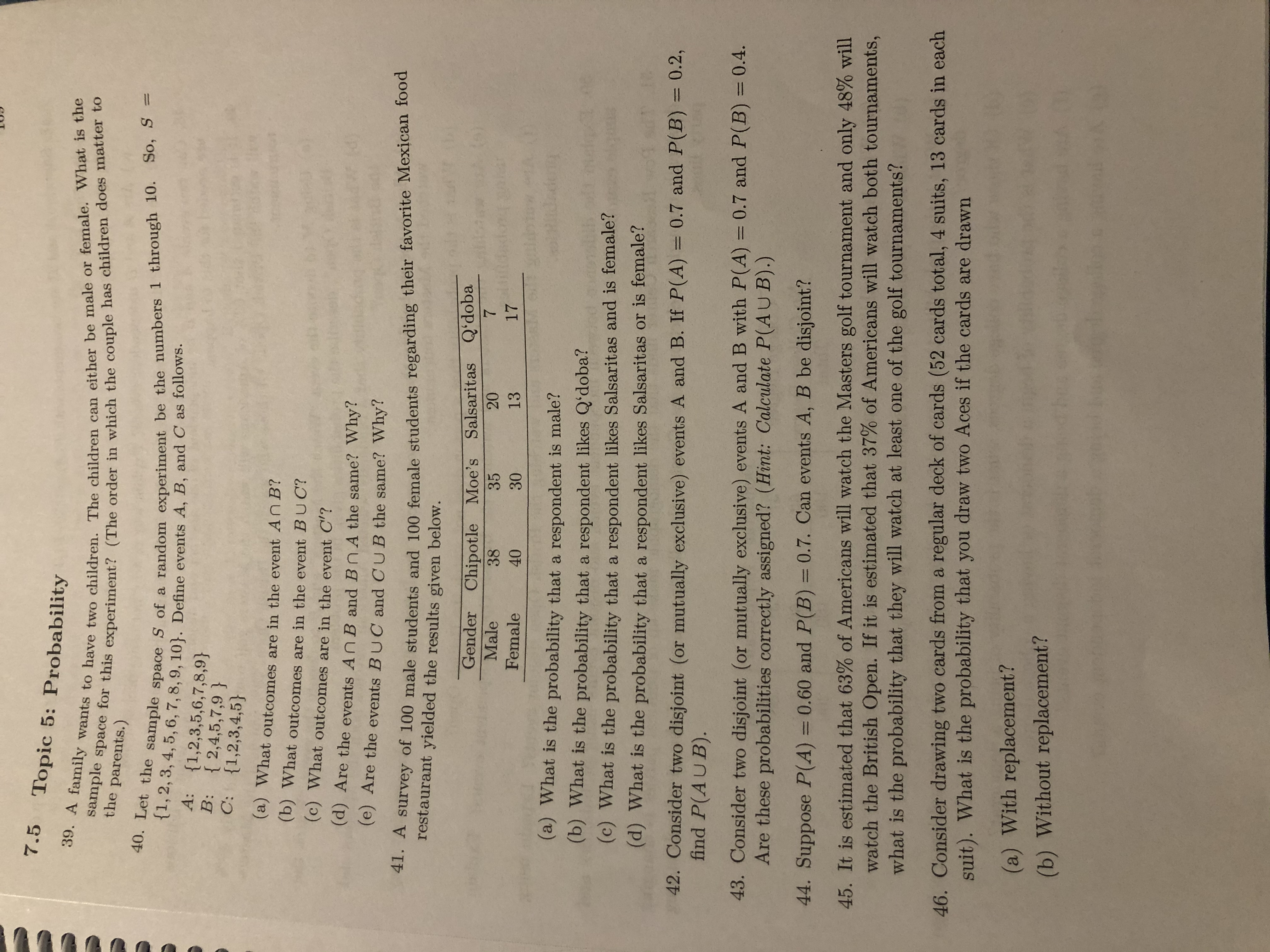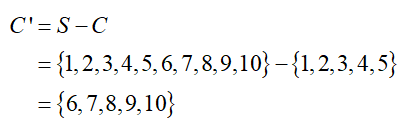# 7.5Topic 5: Probability39. A family wants to have two children. The children can either be male or female. What is thesample space for this experiment? (The order in which the couple has children does matter tothe parents.)40. Let the sample space S of a random experiment be the numbers 1 through 10. So, S ={1,2, 3, 4, 5, 6, 7,8, 9, 10}. Define events A, B, and C as follows.A:{1,2,3,5,6,7,8,9}B: { 2,4,5,7,9 }{1,2,3,4,5}C:(a) What outcomes are in the event AN B?(b) What outcomes are in the event BUC?(c) What outcomes are in the event C'?(d) Are the events An B and BnA the same? Why?(e) Are the events BUC and CUB the same? Why?41. A survey of 100 male students and 100 female students regarding their favorite Mexican foodrestaurant yielded the results given below.Gender Chipotle Moe's Salsaritas QʻdobaMale2035381713Female3040dadeng(a) What is the probability that a respondent is male?(b) What is the probability that a respondent likes Q'doba?(c) What is the probability that a respondent likes Salsaritas and is female?(d) What is the probability that a respondent likes Salsaritas or is female?42. Consider two disjoint (or mutually exclusive) events A and B. If P(A) = 0.7 and P(B) = 0.2,find P(AUB).%3D%3D43. Consider two disjoint (or mutually exclusive) events A and B with P(A) = 0.7 and P(B) = 0.4.Are these probabilities correctly assigned? (Hint: Calculate P(AUB).)%3D%3D44. Suppose P(A) = 0.60 and P(B) = 0.7. Can events A, B be disjoint?%3D%3D45. It is estimated that 63% of Americans will watch the Masters golf tournament and only 48% willwatch the British Open. If it is estimated that 37% of Americans will watch both tournaments,what is the probability that they will watch at least one of the golf tournaments?46. Consider drawing two cards from a regular deck of cards (52 cards total, 4 suits, 13 cards in eachsuit). What is the probability that you draw two Aces if the cards are drawn(a) With replacement?(b) Without replacement?

Question
71 views

Number 40 parts B, C, and Ehelp_outlineImage Transcriptionclose7.5 Topic 5: Probability 39. A family wants to have two children. The children can either be male or female. What is the sample space for this experiment? (The order in which the couple has children does matter to the parents.) 40. Let the sample space S of a random experiment be the numbers 1 through 10. So, S = {1,2, 3, 4, 5, 6, 7,8, 9, 10}. Define events A, B, and C as follows. A: {1,2,3,5,6,7,8,9} B: { 2,4,5,7,9 } {1,2,3,4,5} C: (a) What outcomes are in the event AN B? (b) What outcomes are in the event BUC? (c) What outcomes are in the event C'? (d) Are the events An B and BnA the same? Why? (e) Are the events BUC and CUB the same? Why? 41. A survey of 100 male students and 100 female students regarding their favorite Mexican food restaurant yielded the results given below. Gender Chipotle Moe's Salsaritas Qʻdoba Male 20 35 38 17 13 Female 30 40 dadeng (a) What is the probability that a respondent is male? (b) What is the probability that a respondent likes Q'doba? (c) What is the probability that a respondent likes Salsaritas and is female? (d) What is the probability that a respondent likes Salsaritas or is female? 42. Consider two disjoint (or mutually exclusive) events A and B. If P(A) = 0.7 and P(B) = 0.2, find P(AUB). %3D %3D 43. Consider two disjoint (or mutually exclusive) events A and B with P(A) = 0.7 and P(B) = 0.4. Are these probabilities correctly assigned? (Hint: Calculate P(AUB).) %3D %3D 44. Suppose P(A) = 0.60 and P(B) = 0.7. Can events A, B be disjoint? %3D %3D 45. It is estimated that 63% of Americans will watch the Masters golf tournament and only 48% will watch the British Open. If it is estimated that 37% of Americans will watch both tournaments, what is the probability that they will watch at least one of the golf tournaments? 46. Consider drawing two cards from a regular deck of cards (52 cards total, 4 suits, 13 cards in each suit). What is the probability that you draw two Aces if the cards are drawn (a) With replacement? (b) Without replacement? fullscreen
check_circle

(b) Outcomes of the event B∪C:

Here, A:{1,2,3,4,5,6,7,8,9}, B:{2,4,5,7,9}, C:{1,2,3,4,5}.

Union of B and C is the set of elements in both B and C.

Thus, the outcomes of the event B∪C are {1,2,3,4,5,7,9}

(c) Outcomes in the event C’

The outcomes in the event C’ is obtained as follows:...

### Want to see the full answer?

See Solution

#### Want to see this answer and more?

Solutions are written by subject experts who are available 24/7. Questions are typically answered within 1 hour.*

See Solution
*Response times may vary by subject and question.
Tagged in